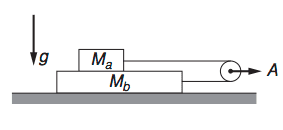# Two blocks and a pulley

## Homework Statement

Mass Ma lies on top of mass Mb, as shown. Assume Mb > Ma. The two blocks are pulled from rest by a massless rope passing over a pulley. The pulley is accelerated at rate A. Block Mb slides on the table without friction, but there is a constant friction force f between Ma and Mb due to their relative motion. Find the tension in the rope.## The Attempt at a Solution

Newton's second law in the y direction for both masses:
$N_1 - M_a g = 0$
$N_2 - N_1 - M_b g = 0$
In the x-direction:
$T_a - F_a = M_a a_a$
$T_b + F_a = M_b a_b$
where
$F_a = \mu M_a g$

Ok, so I suppose we can assume the tension in the rope is the same at every point since it's massless. So $T_a = T_b = T$. Now, the thing is, friction can't cause the blocks to move, it can only oppose motion. So maybe the friction here has to be static friction and we assume the blocks are not moving relative to each other? Otherwise, one of the blocks would have to be moving opposite the direction in which we apply the force, which seems wrong. I'm really not sure where to go with this problem.

I think you are doing alright . In the question it is given that there is relative motion between the blocks , so the friction between the blocks is kinetic in nature . You just take it as 'f' . No need to introduce coefficient of friction.

Apart from the equations you have formed you need one more relation i.e between the acceleration of pulley and the acceleration of the blocks .

I guess that is the part that I am having the most difficulty with. I don't see any way to incorporate the acceleration applied to the pulley. The only thing I can think of is that accelerating the pulley to the right is the same as subjecting the pulley to a gravitational acceleration to the left and holding it in place. Then we could say that the blocks experience a fictitious "force" to the left from this acceleration. But other than that I am stumped.

Look at the picture I have attached .

Let a,b,c be the coordinates of the lower block ,upper block and the pulley respectively.Express the length of the rope in terms of a,b,c .Then use the fact that rope length remains constant . This will give you the relationship between the acceleration of the three entities .

#### Attachments

Last edited:
Oh... ok. So L = (c - a) + (c - b) and differentiating twice, $2A = a_a + a_b$. Solving for T we get $T = 2 \frac{m_a m_b}{m_a + m_b}A + f$

Oh... ok. So L = (c - a) + (c - b) and differentiating twice, $2A = a_a + a_b$

Correct .

Solving for T we get $T = 2 \frac{m_a m_b}{m_a + m_b}A + f$

This doesn't look right .Please recheck.

•ranjhahah
haruspex
Solving for T we get $T = 2 \frac{m_a m_b}{m_a + m_b}A + f$